Begtostudy(白途思)'s Professional Technology Blog

2.1 点估计

2.1.1 无偏性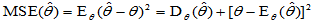（2-1）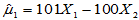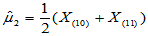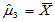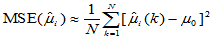N=10000; m=5; n=20;

mse1=0; mse2=0; mse3=0;

for k=1:N

x=chi2rnd(m,1,n);

m1=101*x(1)-100*x(2);

m2=median(x);

m3=mean(x);

mes1=mse1+(m1-m)^2;

mes2=mse2+(m2-m)^2;

mes3=mse3+(m3-m)^2;

end

mse1=mes1/N

mse2=mes2/N

mse3=mes3/N

mse1 =

58.1581

mse2 =

7.8351e-005

mse3 =

9.4469e-006

s=5;

N=10000;

mse1=0; mse2=0;

for k=1:N

x=5.*rand(1,50);

s1=2*mean(x);

s2=max(x);

mse1=mse1+(s1-s)^2;

mse2=mse2+(s2-s)^2;

end

mse1=mse1/N; mse2=mse2/N;

[mse1,mse2]

2.1.2 有效性

2.1.3 相合性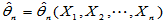为总体未知参数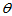的估计量，如果对于任意给定的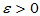，总有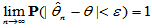（2-2）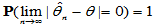（2-3）

2.2 区间估计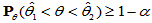2.2.1 单正态总体均值的置信区间

（1）方差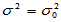已知情形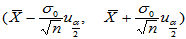（2-4）

1.1, 2.2, 3,3, 4.4, 5.5

x=[1.1,2.2,3.3,4.4,5.5];

n=length(x);

m=mean(x);

c=2.3/sqrt(n);

d=c*norminv(0.975);

a=m-d; b=m+d;

[a,b]

（2）方差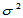未知情形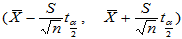（2-4）

S=std(x); dd=S*tinv(0.975,4)/sqrt(n);

aa=m-dd; bb=m+dd; [aa,bb]

2.2.2 单正态总体方差的置信区间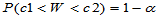的置信区间为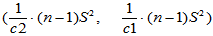（2-5）

c1=chi2inv(0.025,4);

c2=chi2inv(0.975,4);

T=(n-1)*var(x);

aaa=T/c2; bbb=T/c1;

[aaa,bbb]

2.2.3 两正态总体均值差的置信区间

（1）方差已知情形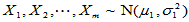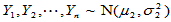，两样本独立，此时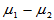的置信区间为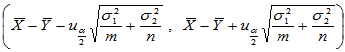（2-6）

（2）方差未知但相等：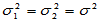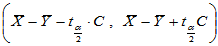（2-7）

2.2.4 两正态总体方差比的置信区间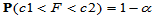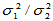的置信区间为：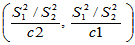（2-7）

A：-0.12 -0.80 -0.05 -0.04 -0.01 0.05 0.07 0.21

B：-1.50 -0.80 -0.40 -0.10 0.20 0.61 0.82 1.24

x=[-0.12,-0.80,-0.05,-0.04,-0.01,0.05,0.07,0.21];

y=[-1.50,-0.80,-0.40,-0.10,0.20,0.61, 0.82,1.24];

v1=var(x); v2=var(y);

c1=finv(0.025,7,7); c2=finv(0.975,7,7);

a=(v1/v2)/c2; b=(v1/v2)/c1; [a,b]Email：begtostudy#gmail.com